# Model theory

The part of mathematical logic studying mathematical models (cf. Model (in logic)).

The origins of model theory go back to the 1920's and 1930's, when the following two fundamental theorems were proved.

## Contents

### Theorem 1

(Gödel compactness theorem). If each finite subcollection of a collection $T$ of propositions in a first-order language is consistent, then the whole collection $T$ is consistent (see ).

### Theorem 2

(Löwenheim–Skolem theorem). If a collection of propositions in a first-order language of signature $\Omega$ has an infinite model, then it has a model of any infinite cardinality not less than the cardinality of $\Omega$.

Theorem 1 has had extensive application in algebra. On the basis of this theorem, A.I. Mal'tsev created a method of proof of local theorems in algebra (see Mal'tsev local theorems).

Let $A$ be an algebraic system of signature $\Omega$, let $|A|$ be the underlying set of $A$, let $X\subseteq|A|$, let $(\Omega,X)$ denote the signature obtained from $\Omega$ by the addition of symbols for distinguished elements $c_a$ for all $a\in X$, and let $(A,X)$ denote the algebraic system of signature $(\Omega,X)$ which is an enrichment of $A$ in which for each $a\in X$ the symbol $c_a$ is interpreted by the element $a$. The set $O(A)$ of all closed formulas of the signature $(\Omega,|A|)$ in a first-order language which are true in the system $(A,|A|)$ is called the elementary diagram of the algebraic system $A$ (or the description of the algebraic system $A$), and the set $D(A)$ of those formulas from $O(A)$ which are either atomic or the negation of an atomic formula is called the diagram of $A$. An algebraic system $B$ is called an elementary extension of $A$ if $|A|\subseteq|B|$ and if $(B,|A|)$ is a model for $O(A)$. In this case $A$ is called an elementary subsystem of $B$. For example, the set of rational numbers with the usual order relation is an elementary subsystem of the system of real numbers with the usual order relation.

A subsystem $A$ of an algebraic system $B$ of signature $\Omega$ is an elementary subsystem of $B$ if and only if for each closed formula $(\exists v)\Phi(v)$ in the first-order language of signature $(\Omega,|A|)$ which is true in $(B,|A|)$ there is an $a\in|A|$ such that $\Phi(c_a)$ is true in $(B,|A|)$. It follows at once from this criterion that the union of an increasing chain of elementary subsystems is an elementary extension of each of these subsystems. If a closed $\forall\exists$-formula in a first-order language is true in every system of an increasing chain of systems, then it is true in the union of the chain (see , ).

Let the signature $\Omega$ contain a one-place relation symbol $U$. One says that a model $A$ of a theory $T$ of signature $\Omega$ has type $(\alpha,\beta)$ if the cardinality of $|A|$ is equal to $\alpha$ and if the cardinality of $U(A)=\{a\in|A|: A\models U(a)\}$ is equal to $\beta$. Vaught's theorem: If an elementary theory $T$ of countable signature has a model of type $(\alpha,\beta)$ where $\alpha>\beta$, then $T$ has a model of type $(\aleph_1,\aleph_0)$ (see , , ). Under the assumption that the generalized continuum hypothesis holds, an elementary theory of countable signature has models of types $(\aleph_{\alpha+2},\aleph_{\alpha+1})$ for each $\alpha$, if it has a model of type $(\aleph_1,\aleph_0)$ (see ). Under the same assumption the theory ${\rm Th}(A)$, where the signature of $A$ is $(+,\,.\,,0,1,<,U)$, $|A|$ is the set of all real numbers, $U(A)$ the set of all integers, and $+,\,.\,,0,1,<$, are defined in the usual way, does not have a model of type $(\aleph_2,\aleph_0)$.

Let $(A,P)$ denote the enrichment of the algebraic system $A$ by a predicate $P$, and let $\Omega,P)$ be the signature obtained from $\Omega$ by the addition of the predicate symbol $P$. In many cases it is important to understand when in each member of a class $\mathcal K$ of algebraic systems of signature $(\Omega,P)$ the predicate $P$ is given by a formula in the first-order language of the signature $\Omega$. A partial answer to this question is given by Beth's definability theorem: There exists a formula $\Phi(x)$ in the first-order language of signature $\Omega$ such that the formula $(\forall x)(\Phi(x)\leftrightarrow P(x))$ is true for all members of an axiomatizable class $\mathcal K$ of signature $(\Omega,P)$ if and only if the set $\{P: (A,P)\in\mathcal K\}$ contains at most one element for each algebraic system $A$ of signature $\Omega$ (see , ).

Much research in model theory is connected with the study of properties preserved under operations on algebraic systems. The most important operations include homomorphism, direct and filtered products.

A statementis said to be stable with respect to homomorphisms if the truth ofin an algebraic systemimplies the truth ofin all epimorphic images of. A formulain a first-order language is called positive ifdoes not contain negation and implication signs. It has been proved (see , ) that a statementin a first-order language is stable relative to homomorphisms if and only ifis equivalent to a positive statement. A similar theorem holds for the language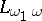.

A formula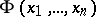in a first-order language of signatureis called a Horn formula it it can be obtained by conjunction and quantification from formulas of the form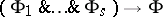,, where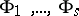are atomic formulas in the first-order language of. Examples of Horn formulas are identities and quasi-identities. Central in the theory of ultraproducts is the theorem of J. Łos: Every formula in a first-order language is stable with respect to any ultrafilter (see ). A formula in a first-order language is conditionally stable with respect to any filter if and only if it is equivalent to a Horn formula. There is the following theorem (see ): Two algebraic systemsandof signatureare elementarily equivalent if and only if there is an ultrafilteron a setsuch thatandare isomorphic. The cardinality of a filtered product is countably infinite if for each natural numberthe number of factors of cardinalityis finite. If for each natural numberthe set of indices for which the corresponding factors have cardinalitydoes not belong to, then the cardinality of the ultraproduct with respect to a non-principal ultrafilteron a countable setis equal to that of the continuum. For each infinite setof cardinalitythere is a filteronsuch that for each filter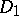oncontaining, and each infinite set, the cardinality of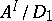is not less than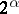(see ).

Many applications have been found for the Ehrenfeucht–Mostowski theorem on the existence of models with a large number of automorphisms (see ): For any totally ordered setin an axiomatizable class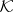of algebraic systems containing an infinite system, there is a systemsuch that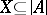and such that each order-preserving one-to-one mapping ofontocan be extended to an automorphism of.

The major notions in model theory are those of universal, homogeneous and saturated systems. Letandbe algebraic systems of a signature. A mappingof a setinto a set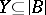is called elementary if for each formulain the first-order language of the signatureand any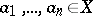the equivalenceholds. A systemis called-universal if for every systemthat is elementarily equivalent toand of cardinality not exceeding, there is an elementary mapping frominto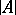. A systemis called-homogeneous if for every setof cardinality less than, every elementary mapping fromintocan be extended to an elementary mapping ofonto(that is, to an automorphism of). A systemof signatureis called-saturated if for every setof cardinality less thanand every collection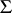of formulas in the first-order language of the signaturenot containing free variables other than,finitely satisfiable in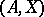implies thatis satisfiable in. A system is called universal (respectively, homogeneous or saturated) ifis-universal (respectively,-homogeneous or-saturated), whereis the cardinality of. A system is saturated if and only if it is simultaneously universal and homogeneous. Two elementary equivalent saturated systems of the same cardinality are isomorphic (see ). All uncountable models of elementary theories which are categorical in uncountable cardinalities (cf. Categoricity in cardinality) and of countable signature are saturated (Morley's theorem, see , ). A large number of examples of-saturated systems is given by ultraproducts. For example, ifis a non-principal ultrafilter on a countable set, thenis an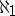-saturated system for any algebraic system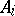(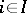) of a countable signature.

The basic problems of model theory are the study of the expressive possibilities of a formalized language and the study of classes of structures defined by means of such a language. Some important properties of stable theories have been found, and the classes of categorical and superstable theories have been studied in even more detail.

The basic apparatus for the study of stable theories is the classification of formulas and locally consistent sets of formulas in these theories. Such a classification can be obtained by means of ascribing to formulas their ranks. Such ranks are usually ordinals and the ranking functions are given with the help of special topologies and other means. The study of ranking functions and their improvements is a rich source of information on the theories.

In the study of classes of models one is concerned with the number of distinct models, up to isomorphism, of a theory of a given cardinality, the existence of special models, for example, simple, minimal, saturated, homogeneous, universal, etc., and one creates means for constructing them.

The classical examples of application of methods of model theory are the papers of A. Robinson and his school, which developed an independent science — non-standard analysis; from the work of Mal'tsev and his school the applications of model-theoretic methods to topological algebra have been developed; the latest results on the properties of stable theories have been used in the study of concrete algebraic questions.

The above problems arose also in the study of various non-elementary languages, for example, obtained by the addition of new quantifiers, the introduction of infinite expressions, modalities, etc.

How to Cite This Entry:
Model theory. Encyclopedia of Mathematics. URL: http://encyclopediaofmath.org/index.php?title=Model_theory&oldid=30757
This article was adapted from an original article by A.D. TaimanovM.A. Taitslin (originator), which appeared in Encyclopedia of Mathematics - ISBN 1402006098. See original article